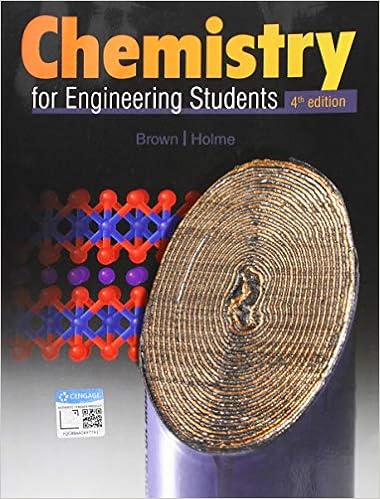# Chem ch 10 review-Chapter 7 The Quantum-Mechanical Model of the Atom Tro.pdf

• Notes
• 16

This preview shows page 1 - 3 out of 16 pages.

##### We have textbook solutions for you!
The document you are viewing contains questions related to this textbook.The document you are viewing contains questions related to this textbook.
Chapter 7 / Exercise 7.56
Chemistry for Engineering Students
Brown/HolmeExpert Verified
1 Chemistry: A Molecular Approach (Tro)Chapter 10 Chemical Bonding II: Molecular Shapes, Valance Bond Theory, and Molecular Orbital Theory 1) Determine the electron geometry (eg) and molecular geometry(mg) of BCl3. A) eg=trigonal planar, mg=trigonal planar B) eg=tetrahedral, mg=trigonal planar C) eg=tetrahedral, mg=trigonal pyramidal D) eg=trigonal planar, mg=bent E) eg=trigonal bipyramidal, mg=trigonal bipyramidal Answer:
A 2) Determine the electron geometry (eg), molecular geometry (mg), and polarity of SO2.
B 3) Determine the electron geometry (eg), molecular geometry (mg), and polarity of SO3.
C 4) Determine the electron geometry (eg) and molecular geometry (mg) of CO32°.
D 5) Determine the electron geometry (eg) and molecular geometry (mg) of SiF4. A) eg=tetrahedral, mg=trigonal pyramidal B) eg=octahedral, mg=square planar C) eg=trigonal bipyramidal, mg=trigonal pyramidal D) eg=tetrahedral, mg=bent E) eg=tetrahedral, mg=tetrahedral Answer:
E
##### We have textbook solutions for you!
The document you are viewing contains questions related to this textbook.The document you are viewing contains questions related to this textbook.
Chapter 7 / Exercise 7.56
Chemistry for Engineering Students
Brown/HolmeExpert Verified
2 6) Determine the electron geometry (eg) and molecular geometry (mg) of PF5.
7) Determine the electron geometry (eg) and molecular geometry (mg) of BrF3.
B 8) Determine the electron geometry (eg) and molecular geometry (mg) of ICl2°.
C
•••